# Excel如果if函数有多个条件，应该怎么使用

Excel中的IF函数，如果需要满足多个条件，那么应该怎么使用if函数，怎么填写呢?

if函数是 Excel 中的条件判断函数，它由条件与两个返回结果组成，当条件成立时，返回真，否则返回假。if函数中的条件既可以单条件，也可以是多条件;多条件组合有三种方式，一种为多个 if 嵌套，第二种为用 And(或 *)组合多个条件，第三种为用 Or(或 +)组合多个条件。用 And(或 *)组合条件是与的关系，用 Or(或 +)组合条件是或的关系，它们的写法比 if 嵌套简单。以下就是它们的具体操作方法。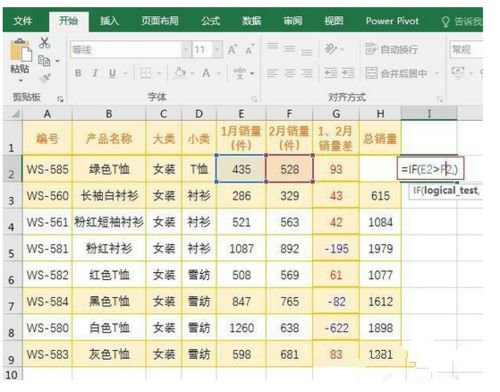Excel if函数语法

1

一、Excel if函数语法1、表达式：IF(logical_test,[value_if_true],[value_if_false])中文表达式：如果(条件，条件为真时执行的操作，条件为假时执行的操作)2、说明：[value_if_true] 和 [value_if_false] 表示可选项，即它们可以不写，如图1所示：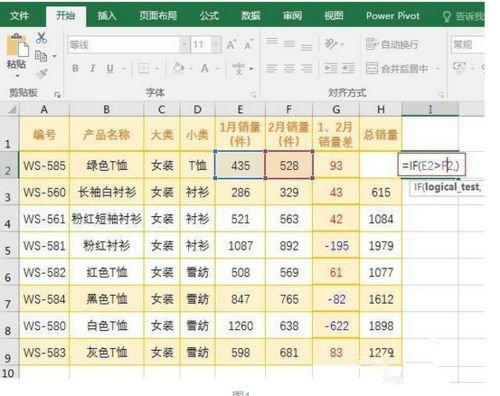2

按回车，返回 False，因为 E2 为 435，F2 为 528，E2 F2 不成立，如图2所示：图2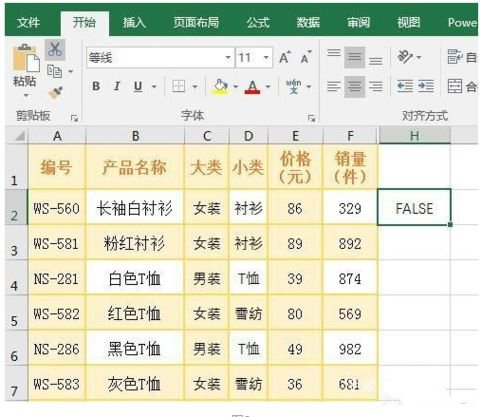3

另外，=IF(3 2,)，返回 0，此处 0 表示假。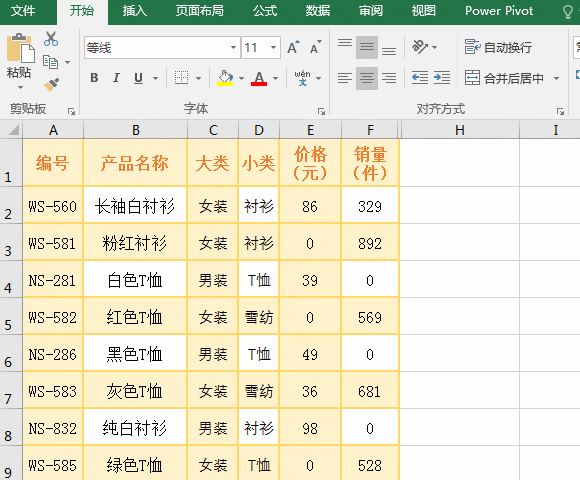Excel if函数单条件使用方法

1

一个服装销量表中，价格为0的表示已下架，否则表示正在出售，假如要把它们分别用下架和出售中标识出来，操作过程步骤，如图3所示2

操作过程步骤说明：

选中 H2 单元格，输入公式 =IF(E2=0,下架,出售中)，按回车，则返回出售中;把鼠标移到 H2 右下角的单元格填充柄上，按住左键并往下拖，则所经过单元格全填充为出售中，按 Ctrl + S 保存，则价格为 0 的单元格用下架填充，其它单元格用出售中填充。

公式说明：公式 =IF(E2=0,下架,出售中) 中，E2=0 为条件，当条件为真时，返回下架，否同返回出售中。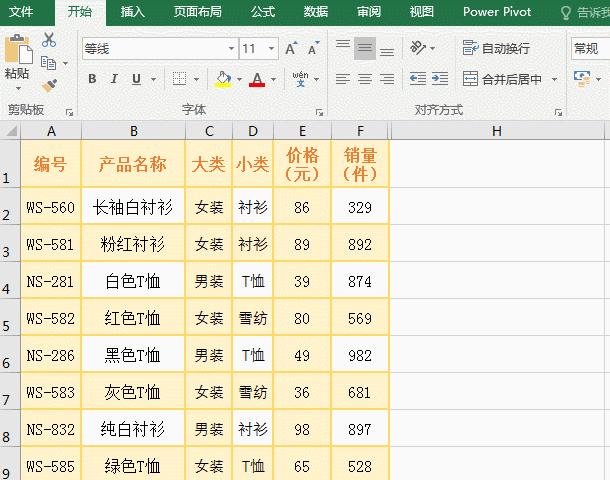Excel if函数嵌套多条件使用方法

1

假如要标出服装销量表中，大类为女装、价格大于等于 80 且销量大于 800 的服装，操作过程步骤，如图4所示2

操作过程步骤说明：

选中 H2 单元格，把公式 =IF(C2=女装,IF(E2=80,IF(F2800,满足条件,不满足条件),不满足条件),不满足条件) 复制到 H2，按回车，则返回不满足条件;再次选中 H2，把鼠标移到 H2 的单元格填充柄上，按住左键并往下拖，则所经过单元格用不满足条件填充，按 Ctrl + S 保存，同样 H3 用满足条件填充，其它单元格仍用不满足条件填充。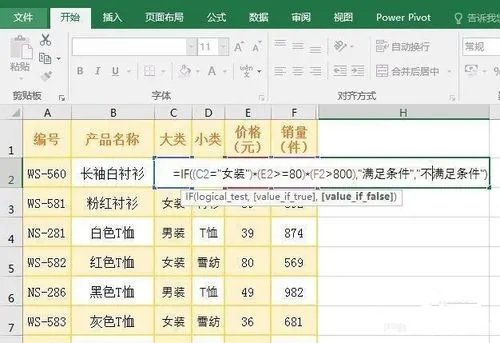3

公式说明：

=IF(C2=女装,IF(E2=80,IF(F2800,满足条件,不满足条件),不满足条件),不满足条件)

由三个 if 组成，即在一个 if 中嵌套了两个 if。第一个 if 的条件为 C2=女装，如果条件为真，则执行 IF(E2=80,IF(F2800,满足条件,不满足条件),不满足条件);否则返回不满足条件。第二个 if 的条件为 E2=80，如果条件为真，则执行 IF(F2800,满足条件,不满足条件)，否则返回不满足条件。第三个 if 的条件为 F2800，如果条件为真，返回满足条件，否则返回不满足条件

提示：

if 最多只能嵌套 64 个 if，尽管如此，在写公式过程中，尽量少嵌套 if;

一方面便于阅读与修改，另一方面执行效率也高一些。

Excel if函数用 And 与 OR 组合多个条件使用方法

1

用 And 组合多个条件，为与的关系

把上例中的多 if 嵌套公式 =IF(C2=女装,IF(E2=80,IF(F2800,满足条件,不满足条件),不满足条件),不满足条件) 改为用 And 组合，操作过程步骤，如图5所示：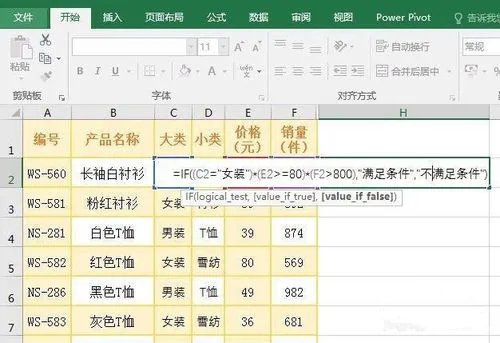2

操作过程步骤说明：

选中 H2 单元格，把公式 =IF(AND(C2=女装,E2=80,F2800),满足条件,不满足条件) 复制到 H2，按回车，则返回不满足条件;

同样往下拖并保存，返回跟上例一样的结果，说明公式正确。

3

公式说明：

=IF(AND(C2=女装,E2=80,F2800),满足条件,不满足条件)

公式用 And 函数组合了三个条件，分别为 C2=女装,E2=80,F2800，当同时满足三个条件时(即 AND(C2=女装,E2=80,F2800) 返回真)，返回满足条件，否则返回不满足条件。

4

用 * 代替 And

A、把公式

=IF(AND(C2=女装,E2=80,F2800),满足条件,不满足条件)

用 * 代替 And 后变为：

=IF((C2=女装)*(E2=80)*(F2800),满足条件,不满足条件)

B、按回车，返回不满足条件，往下拖保存后，也是返回一样的结果。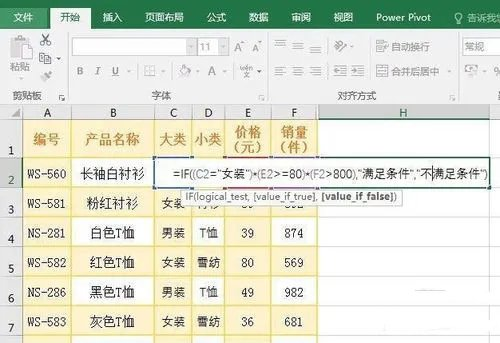5

用 Or 组合多个条件，为或的关系

1、把上例中的 And 组合多个条件公式 =IF(AND(C2=女装,E2=80,F2800),满足条件,不满足条件) 改为用 Or 组合，操作过程步骤，如图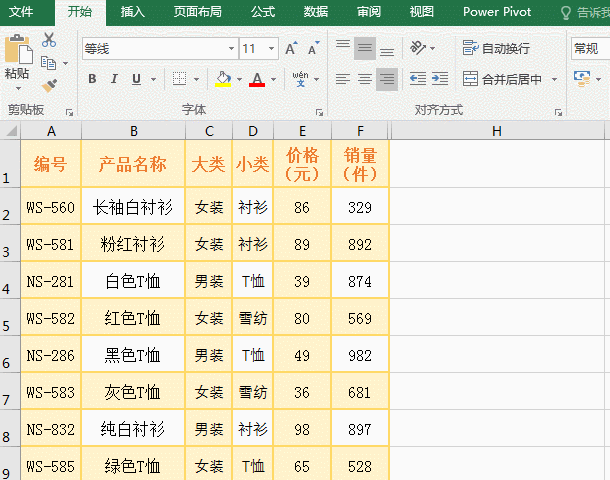6

2、操作过程步骤说明：选中 H2 单元格，把公式 =IF(OR(C2=女装,E2=80,F2800),满足条件,不满足条件) 复制到 H2，按回车，则返回满足条件;同样往下拖并保存，全部返回满足条件。

3、公式说明：

=IF(OR(C2=女装,E2=80,F2800),满足条件,不满足条件)

公式用 Or 函数组合了三个条件，分别为 C2=女装,E2=80,F2800，即 OR(C2=女装,E2=80,F2800)，

意思是：只要满足一个条件，就返回真;一条件都不满足才返回假。演示中，每条记录都满足一个条件，所以全返回满足条件。

7

4、用 + 代替 Or

A、把公式

=IF(OR(C2=女装,E2=80,F2800),满足条件,不满足条件)

用 + 代替 Or 后变为：

=IF((C2=女装)+(E2=80)+(F2800),满足条件,不满足条件)

如图8所示：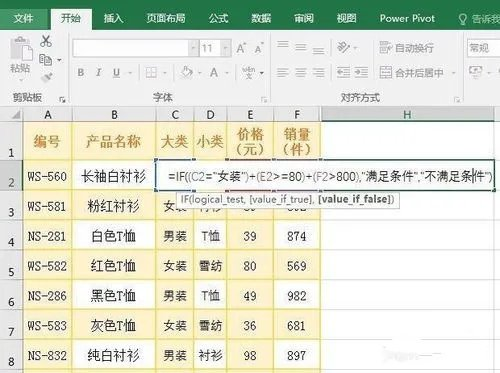8

B、按回车，返回满足条件，往下拖保存后，也是全部返回满足条件，说明公式正确。